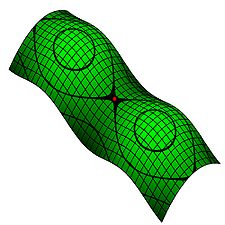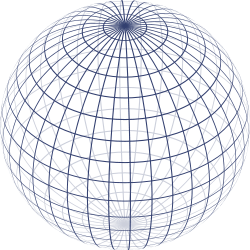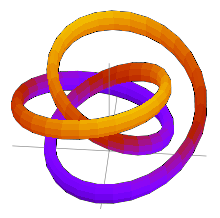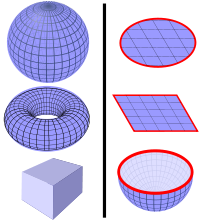# Surface

SurfaceAn open surface with X-, Y-, and Z-contours shown.

In mathematics, specifically in topology, a surface is a two-dimensional topological manifold. The most familiar examples are those that arise as the boundaries of solid objects in ordinary three-dimensional Euclidean space R3 — for example, the surface of a ball. On the other hand, there are surfaces, such as the Klein bottle, that cannot be embedded in three-dimensional Euclidean space without introducing singularities or self-intersections.

To say that a surface is "two-dimensional" means that, about each point, there is a coordinate patch on which a two-dimensional coordinate system is defined. For example, the surface of the Earth is (ideally) a two-dimensional sphere, and latitude and longitude provide two-dimensional coordinates on it (except at the poles and along the 180th meridian).

The concept of surface finds application in physics, engineering, computer graphics, and many other disciplines, primarily in representing the surfaces of physical objects. For example, in analyzing the aerodynamic properties of an airplane, the central consideration is the flow of air along its surface.

## Definitions and first examples

A (topological) surface is a nonempty second countable Hausdorff topological space in which every point has an open neighbourhood homeomorphic to some open subset of the Euclidean plane E2. Such a neighborhood, together with the corresponding homeomorphism, is known as a (coordinate) chart. It is through this chart that the neighborhood inherits the standard coordinates on the Euclidean plane. These coordinates are known as local coordinates and these homeomorphisms lead us to describe surfaces as being locally Euclidean.

More generally, a (topological) surface with boundary is a Hausdorff topological space in which every point has an open neighbourhood homeomorphic to some open subset of the upper half-plane H2. These homeomorphisms are also known as (coordinate) charts. The boundary of the upper half-plane is the x-axis. A point on the surface mapped via a chart to the x-axis is termed a boundary point. The collection of such points is known as the boundary of the surface which is necessarily a one-manifold, that is, the union of closed curves. On the other hand, a point mapped to above the x-axis is an interior point. The collection of interior points is the interior of the surface which is always non-empty. The closed disk is a simple example of a surface with boundary. The boundary of the disc is a circle.

The term surface used without qualification refers to surfaces without boundary. In particular, a surface with empty boundary is a surface in the usual sense. A surface with empty boundary which is compact is known as a 'closed' surface. The two-dimensional sphere, the two-dimensional torus, and the real projective plane are examples of closed surfaces.

The Möbius strip is a surface with only one "side". In general, a surface is said to be orientable if it does not contain a homeomorphic copy of the Möbius strip; intuitively, it has two distinct "sides". For example, the sphere and torus are orientable, while the real projective plane is not (because deleting a point or disk from the real projective plane produces the Möbius strip).

In differential and algebraic geometry, extra structure is added upon the topology of the surface. This added structures detects singularities, such as self-intersections and cusps, that cannot be described solely in terms of the underlying topology.

## Extrinsically defined surfaces and embeddingsA sphere can be defined parametrically (by x = r sin θ cos φ, y = r sin θ sin φ, z = r cos θ) or implicitly (by x² + y² + z² − r² = 0.)

Historically, surfaces were initially defined as subspaces of Euclidean spaces. Often, these surfaces were the locus of zeros of certain functions, usually polynomial functions. Such a definition considered the surface as part of a larger (Euclidean) space, and as such was termed extrinsic.

In the previous section, a surface is defined as a topological space with certain property, namely Hausdorff and locally Euclidean. This topological space is not considered as being a subspace of another space. In this sense, the definition given above, which is the definition that mathematicians use at present, is intrinsic.

A surface defined as intrinsic is not required to satisfy the added constraint of being a subspace of Euclidean space. It seems possible at first glance that there are surfaces defined intrinsically that are not surfaces in the extrinsic sense. However, the Whitney embedding theorem asserts that every surface can in fact be embedded homeomorphically into Euclidean space, in fact into E4. Therefore the extrinsic and intrinsic approaches turn out to be equivalent.

In fact, any compact surface that is either orientable or has a boundary can be embedded in E³; on the other hand, the real projective plane, which is compact, non-orientable and without boundary, cannot be embedded into E³ (see Gramain). Steiner surfaces, including Boy's surface, the Roman surface and the cross-cap, are immersions of the real projective plane into E³. These surfaces are singular where the immersions intersect themselves.

The Alexander horned sphere is a well-known pathological embedding of the two-sphere into the three-sphere.A knotted torus.

The chosen embedding (if any) of a surface into another space is regarded as extrinsic information; it is not essential to the surface itself. For example, a torus can be embedded into E³ in the "standard" manner (that looks like a bagel) or in a knotted manner (see figure). The two embedded tori are homeomorphic but not isotopic; they are topologically equivalent, but their embeddings are not.

The image of a continuous, injective function from R2 to higher-dimensional Rn is said to be a parametric surface. Such an image is so-called because the x- and y- directions of the domain R2 are 2 variables that parametrize the image. Be careful that a parametric surface need not be a topological surface. A surface of revolution can be viewed as a special kind of parametric surface.

If f is a smooth function from R³ to R whose gradient is nowhere zero, Then the locus of zeros of f does define a surface, known as an implicit surface. If the condition of non-vanishing gradient is dropped then the zero locus may develop singularities.

## Construction from polygons

Each closed surface can be constructed from an oriented polygon with an even number of sides, called a fundamental polygon of the surface, by pairwise identification of its edges. For example, in each polygon below, attaching the sides with matching labels (A with A, B with B), so that the arrows point in the same direction, yields the indicated surface.

Any fundamental polygon can be written symbolically as follows. Begin at any vertex, and proceed around the perimeter of the polygon in either direction until returning to the starting vertex. During this traversal, record the label on each edge in order, with an exponent of -1 if the edge points opposite to the direction of traversal. The four models above, when traversed clockwise starting at the upper left, yield

• sphere: ABB − 1A − 1
• real projective plane: ABAB
• torus: ABA − 1B − 1
• Klein bottle: ABAB − 1.

Note that the sphere and the projective plane can both be realized as quotients of the 2-gon, while the torus and Klein bottle require a 4-gon (square).

The expression thus derived from a fundamental polygon of a surface turns out to be the sole relation in a presentation of the fundamental group of the surface with the polygon edge labels as generators. This is a consequence of the Seifert–van Kampen theorem.

Gluing edges of polygons is a special kind of quotient space process. The quotient concept can be applied in greater generality to produce new or alternative constructions of surfaces. For example, the real projective plane can be obtained as the quotient of the sphere by identifying all pairs of opposite points on the sphere. Another example of a quotient is the connected sum.

## Connected sums

The connected sum of two surfaces M and N, denoted M # N, is obtained by removing a disk from each of them and gluing them along the boundary components that result. The boundary of a disk is a circle, so these boundary components are circles. The Euler characteristic χ of M # N is the sum of the Euler characteristics of the summands, minus two:$\chi(M \# N) = \chi(M) + \chi(N) - 2.\,$

The sphere S is an identity element for the connected sum, meaning that S # M = M. This is because deleting a disk from the sphere leaves a disk, which simply replaces the disk deleted from M upon gluing.

Connected summation with the torus T is also described as attaching a "handle" to the other summand M. If M is orientable, then so is T # M. The connected sum is associative, so the connected sum of a finite collection of surfaces is well-defined.

The connected sum of two real projective planes, P # P, is the Klein bottle K. The connected sum of the real projective plane and the Klein bottle is homeomorphic to the connected sum of the real projective plane with the torus; in a formula, P # K = P # T. Thus, the connected sum of three real projective planes is homeomorphic to the connected sum of the real projective plane with the torus. Any connected sum involving a real projective plane is nonorientable.

## Closed surfaces

A closed surface is a surface that is compact and without boundary. Examples are spaces like the sphere, the torus and the Klein bottle. Examples of non-closed surfaces are: an open disk, which is a sphere with a puncture; a cylinder, which is a sphere with two punctures; and the Möbius strip.

### Classification of closed surfacesSome examples of orientable closed surfaces (left) and surfaces with boundary (right). Left: Some orientable closed surfaces are the surface of a sphere, the surface of a torus, and the surface of a cube. (The cube and the sphere are topologically equivalent to each other.) Right: Some surfaces with boundary are the disk surface, square surface, and hemisphere surface. The boundaries are shown in red. All three of these are topologically equivalent to each other.

The classification theorem of closed surfaces states that any connected closed surface is homeomorphic to some member of one of these three families:

1. the sphere;
2. the connected sum of g tori, for$g \geq 1$;
3. the connected sum of k real projective planes, for$k \geq 1$.

The surfaces in the first two families are orientable. It is convenient to combine the two families by regarding the sphere as the connected sum of 0 tori. The number g of tori involved is called the genus of the surface. The sphere and the torus have Euler characteristics 2 and 0, respectively, and in general the Euler characteristic of the connected sum of g tori is 2 − 2g.

The surfaces in the third family are nonorientable. The Euler characteristic of the real projective plane is 1, and in general the Euler characteristic of the connected sum of k of them is 2 − k.

It follows that a closed surface is determined, up to homeomorphism, by two pieces of information: its Euler characteristic, and whether it is orientable or not. In other words, Euler characteristic and orientability completely classify closed surfaces up to homeomorphism.

For closed surfaces with multiple connected components, they are classified by the class of each of their connected components, and thus one generally assumes that the surface is connected.

### Monoid structure

Relating this classification to connected sums, the closed surfaces up to homeomorphism form a monoid with respect to the connected sum, as indeed do manifolds of any fixed dimension. The identity is the sphere, while the real projective plane and the torus generate this monoid, with a single relation P # P # P = P # T, which may also be written P # K = P # T, since K = P # P. This relation is sometimes known as Dyck's theorem after Walther von Dyck, who proved it in (Dyck 1888), and the triple cross surface P # P # P is accordingly called Dyck's surface.

Geometrically, connect-sum with a torus (# T) adds a handle with both ends attached to the same side of the surface, while connect-sum with a Klein bottle (# K) adds a handle with the two ends attached to opposite sides of the surface; in the presence of a projective plane (# P), the surface is not orientable (there is no notion of side), so there is no difference between attaching a torus and attaching a Klein bottle, which explains the relation.

### Surfaces with boundary

Compact surfaces, possibly with boundary, are simply closed surfaces with a number of holes (open discs that have been removed); thus a connected compact surface is classified by the number of boundary components and the class of the corresponding closed surface – equivalently, by the number of boundary components, the orientability, and Euler characteristic. The genus of a compact surface is defined as the genus of the corresponding closed surface.

This follows quickly but not without note from the result for closed surfaces: removing an open disc from a closed surface yields a compact surface with a circle for boundary component, and removing k open discs yields a compact surface with k disjoint circles for boundary components; the locations of the holes is irrelevant because the homeomorphism group acts k-transitively on any connected manifold of dimension at least 2.

Conversely, the boundary of a compact surface is a closed 1-manifold, which, by classification of 1-manifolds (closed curves), is a disjoint union of a finite number of circles, and these circles can be filled in (formally, taking the cone), yielding a closed surface.

Compact orientable surfaces of genus g and with k boundary components are often denote as Σg,k, for example in the study of the mapping class group.

### Riemann surfaces

A closely related example to the classification of compact 2-manifolds is the classification of compact Riemann surfaces, i.e., compact complex 1-manifolds. (Note that the 2-sphere, and the tori are all complex manifolds, in fact algebraic varieties.) Since every complex manifold is orientable, the connected sums of projective planes do not qualify. Thus compact Riemann surfaces are characterized topologically simply by their genus. The genus counts the number of holes in the manifold: the sphere has genus 0, the one-holed torus genus 1, etc.

### Non-compact surfaces

Non-compact surfaces are more difficult to classify; one simple case is puncturing (removing a point, or multiple points) from a closed manifold, but even a question such as the open subsets of the plane is very complicated — for example, the complement of the Cantor set is a non-compact surface. Another example of a non-compact surface is Jacob's ladder, which looks like a ladder made of handlebodies.

### Proof

The classification of closed surfaces has been known since the 1860s, and today a number of proofs exist.

Topological and combinatorial proofs in general rely on the difficult result that every compact 2-manifold is homeomorphic to a simplicial complex, which is of interest in its own right. The most common proof of the classification is (Seifert & Threlfall 1934), which brings every triangulated surface to a standard form. A simplified proof, which avoids a standard form, was discovered by John H. Conway circa 1992, which he called the "Zero Irrelevancy Proof" or "ZIP proof"[note 1] and is presented in (Francis & Weeks 1999).

A geometric proof, which yields a stronger geometric result, is the uniformization theorem. This was originally proven only for Riemann surfaces in the 1880s and 1900s by Felix Klein, Paul Koebe, and Henri Poincaré.

## Surfaces in geometry

Polyhedra, such as the boundary of a cube, are among the first surfaces encountered in geometry. It is also possible to define smooth surfaces, in which each point has a neighborhood diffeomorphic to some open set in E². This elaboration allows calculus to be applied to surfaces to prove many results.

Two smooth surfaces are diffeomorphic if and only if they are homeomorphic. (The analogous result does not hold for higher-dimensional manifolds.) Thus closed surfaces are classified up to diffeomorphism by their Euler characteristic and orientability.

Smooth surfaces equipped with Riemannian metrics are of fundational importance in differential geometry. A Riemannian metric endows a surface with notions of geodesic, distance, angle, and area. It also gives rise to Gaussian curvature, which describes how curved or bent the surface is at each point. Curvature is a rigid, geometric property, in that it is not preserved by general diffeomorphisms of the surface. However, the famous Gauss-Bonnet theorem for closed surfaces states that the integral of the Gaussian curvature K over the entire surface S is determined by the Euler characteristic:$\int_S K \; dA = 2 \pi \chi(S).$

This result exemplifies the deep relationship between the geometry and topology of surfaces (and, to a lesser extent, higher-dimensional manifolds).

Another way in which surfaces arise in geometry is by passing into the complex domain. A complex one-manifold is a smooth oriented surface, also called a Riemann surface. Any complex nonsingular algebraic curve viewed as a complex manifold is a Riemann surface.

Every closed orientable surface admits a complex structure. Complex structures on a closed oriented surface correspond to conformal equivalence classes of Riemannian metrics on the surface. One version of the uniformization theorem (due to Poincaré) states that any Riemannian metric on an oriented, closed surface is conformally equivalent to an essentially unique metric of constant curvature. This provides a starting point for one of the approaches to Teichmüller theory, which provides a finer classification of Riemann surfaces than the topological one by Euler characteristic alone.

A complex surface is a complex two-manifold and thus a real four-manifold; it is not a surface in the sense of this article. Neither are algebraic curves defined over fields other than the complex numbers, nor are algebraic surfaces defined over fields other than the real numbers.

## See also

Wikimedia Foundation. 2010.

Synonyms:

### Look at other dictionaries:

• surface — [ syrfas ] n. f. • 1611; superface 1521; lat. superficies → superficie; de sur et face 1 ♦ Partie extérieure (d un corps), qui le limite en tous sens. ⇒ face. La surface de la Terre, la surface terrestre. À la surface du sol. « une surface pure… …   Encyclopédie Universelle

• Surface — Sur face , n. [F. See {Sur }, and {Face}, and cf. {Superficial}.] 1. The exterior part of anything that has length and breadth; one of the limits that bound a solid, esp. the upper face; superficies; the outside; as, the surface of the earth; the …   The Collaborative International Dictionary of English

• surface — [sʉr′fis] n. [Fr < sur (see SUR 1) + face,FACE, based on L superficies] 1. a) the outer face, or exterior, of an object b) any of the faces of a solid c) the area or extent of such a face …   English World dictionary

• surface — 1610s, from Fr. surface outermost boundary of anything, outside part (16c.), from O.Fr. sur above + face (see FACE (Cf. face)). Patterned on L. superficies surface (see SUPERFICIAL (Cf. superficial)). The verb meaning come to the surface is first …   Etymology dictionary

• surface — ► NOUN 1) the outside part or uppermost layer of something. 2) the upper limit of a body of liquid. 3) outward appearance as distinct from less obvious aspects. ► ADJECTIVE 1) relating to or occurring on the surface. 2) (of transportation) by sea …   English terms dictionary

• Surface — Sur face, v. t. [imp. & p. p. {Surfaced}; p. pr. & vb. n. {Surfacing}.] 1. To give a surface to; especially, to cause to have a smooth or plain surface; to make smooth or plain. [1913 Webster] 2. To work over the surface or soil of, as ground, in …   The Collaborative International Dictionary of English

• surface — [adj] external apparent, covering, depthless, exterior, facial, outer, outside, outward, shallow, shoal, superficial, top; concepts 485,583 Ant. central, core, inside, interior, middle surface [n] external part of something area, cover, covering …   New thesaurus

• surface — Surface. subst. f. L exterieur, le dehors d un corps. Surface platte, unie, raboteuse. la surface de la terre …   Dictionnaire de l'Académie française

• Surface — Sur face, v. i. 1. To rise from the depths of a liquid to the surface; as, the submarine surfaced to recharge its batteries. [PJC] 2. To become known or public; said of information. [PJC] 3. To show up, as a person who was in hiding; as, he… …   The Collaborative International Dictionary of English

• Surface — (fr., spr. Sürfahs), die Oberfläche …   Pierer's Universal-Lexikon

• surface — index bare, cursory, dimension, emerge, issue (send forth), ostensible, side, superficial …   Law dictionary

### Share the article and excerpts

##### Direct link
Do a right-click on the link above
and select “Copy Link”